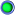Thread: New Jsfx: AnalogSum View Single Post01-19-2019, 06:01 PM #41 sai'ke Human being with feelings   Join Date: Aug 2009 Location: Germoney Posts: 326Whoops, yeah, sorry, I chose epsilon too high. One annoying thing with the method is that it contains a division by a potentially small number (this happens when two subsequent samples have very little difference). To avoid this, you have to replace the solution at that location with what's in the limit there (which can actually be determined analytically). The good part is that the function is relatively well behaved analytically there. The bad part is that it obviously isn't numerically. Anyways, you have to choose this cutoff point (eps in the code) where you insert the limit solution. I chose it a bit too high. The second issue is a precision issue and cannot be resolved afaik. But when you're pushing it that hard, it's hardly being used as a normal saturator anymore. One solution that may be worth investigating is using some sort of lookup table rather than computing the function. Then it can just once be calculated reliably, and numerical issues might not be a thing. Would probably cost some performance tho. Code: ```DO NOT USE THIS VERSION, BETTER VERSION IN LATER POST. desc:Tanh Saturation with anti aliasing tags: saturation distortion anti-aliased version: 1.01 author: Joep Vanlier changelog: + Tweaked epsilon + Tweaked epsilon license: MIT Uses technique from: Parker et al, "REDUCING THE ALIASING OF NONLINEAR WAVESHAPING USING CONTINUOUS-TIME CONVOLUTION", Proceedings of the 19th International Conference on Digital Audio Effects (DAFx-16), Brno, Czech Republic, September 5–9, 2016 I have only implemented the rect version, since the linear one depends on Li2 and LUTs aren't so fast in JSFX. in_pin:left input in_pin:right input out_pin:left output out_pin:right output slider1:0<-6,24,1>Gain (dB) slider2:0<-18,0,1>Ceiling (dB) slider3:1<0,1,1>Antialias? slider4:0<0,1,1>Fix DC? @init bpos=0; @slider preamp = 10^(slider1/20); ceiling = 10^(-slider2/20); inv_ceiling = 10^(slider2/20); @block blah+=samplesblock; @sample spl0=spl0; spl1=spl1; @sample function F0(x, em2x) local() global() instance() ( x - log(2/(1 + em2x)) ); function tanh_prec(x, em2x) local() global() instance() ( (2/(1+em2x))-1 ); function tanh(x) local() global() instance() ( (2/(1+exp(-2*x)))-1 ); function antialiased_tanh_rect(x) local(eps, em2x, F0_xn, diff, diffy) global(slider4) instance(antialias, F0_xnm1, xnm1) ( em2x = exp(-2*x); F0_xn = F0(x, em2x); diff = ( x - xnm1 ); eps = 0.000000000000000001; antialias = (abs(diff) > eps) ? ( F0_xn - F0_xnm1 ) / diff : tanh_prec(.5*(x+xnm1), em2x); F0_xnm1 = F0_xn; xnm1 = x; antialias ); function fix_dc(x) local() global() instance(DC_fixed, prev) ( DC_fixed=0.999*DC_fixed + x - prev; prev=x; DC_fixed ); spl0 *= preamp; spl1 *= preamp; spl0 *= ceiling; spl1 *= ceiling; slider3 ? ( spl0 = ch0.antialiased_tanh_rect(spl0); spl1 = ch1.antialiased_tanh_rect(spl1); ) : ( spl0 = tanh(spl0); spl1 = tanh(spl1); ); slider4 ? ( spl0 = dc0.fix_dc(spl0); spl1 = dc1.fix_dc(spl1); ); spl0 *= inv_ceiling; spl1 *= inv_ceiling;``` __________________ [Tunes] | [Tracker Plugin: Thread|Github|Reapack] | [Routing Plugin: Thread|Github|Reapack] | [Filther: Thread|Github|Reapack] Last edited by sai'ke; 01-20-2019 at 05:23 AM.